# Perimeter and Area Formulas for two dimensional geometrical figures

In this page provided formulas of Perimeter and Area for two dimensional geometrical figures like square, rectangle, Rhombus, parallelogram, trapezium or trapezoid, triangle, right angle triangle, ellipse, circle, sector of a circle, segment of a circle etc

## Formulas for Two Dimensional Geometrical Figures

### Square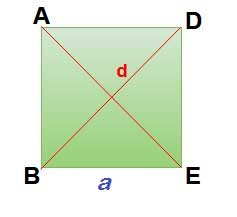Side for square = a  &  Length of diagonal = d

Area of the square = A = side2  = a = (1/2) d2

Perimeter of the square = P = 4 × side = 4 a

Length of diagonal = d =### Rectangle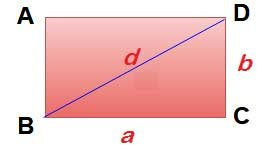Perimeter of the rectangle = 2 × (length + width) = 2 (a + b)

Area of the rectangle = length × width = ab

Length of diagonal  ( d )   = √ (a2 + b2)

### Rhombus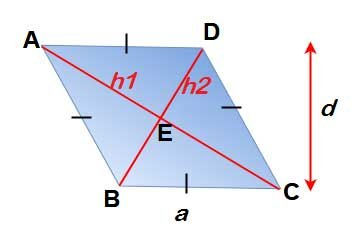For Rhombus all sides are equal = a, Vertical Height = d &  h1, h2 are the diagonals  also  AB || DC , AD || BC

Perimeter of rhombus = 4a

Area of the Rhombus = ad = (1/2) d1 d2

### Parallelogram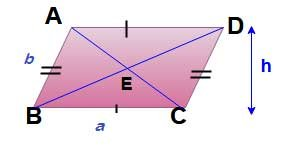Area of  parallelogram = base × height = a x h

Perimeter of  parallelogram = 2 × (side1 + side2) = 2 ( a + b)

### Trapezium (Trapezoid)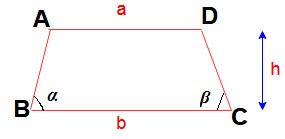In trapezium  two sides are in parallel,

Area of  Trapezium  or Trapezoid =Perimeter of Trapezium  or Trapezoid =### Triangle

####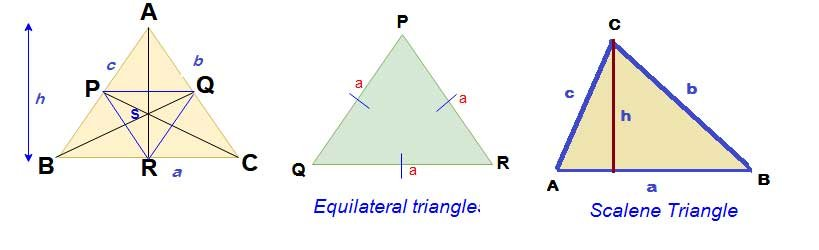Formulas for triangle

Area of the triangle = (1/2) x Base x Height =Area of the triangle =Where Semi perimeter =Radius of incircle triangle = Area /S

#### Formulas for equilateral triangle

Perimeter of the equilateral triangle = 3 x Side = 3a

Area of the equilateral triangle  == 0.433 x (side) 2

Radius of circumference circle of an equilateral triangle =Area of circumference circle of an equilateral triangle =Radius of incircle of an equilateral triangle ==a / (2 √3)

###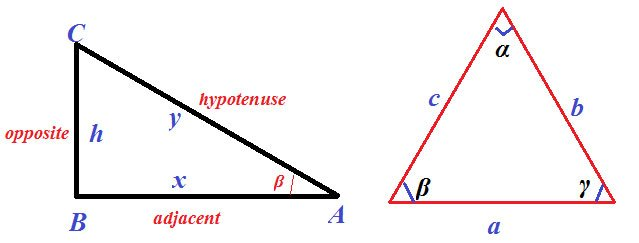In above figure ABC is a right angle triangle x = length of adjacent side, y = length of hypotenuse & h = height of the triangle.

Pythagoras’ Law –Area of the right angle triangle =Sin β = h / y  ⇒  h  = y  Sin β

Cos β = x / y  ⇒  x  = y  Cos β

Tan β = h / x ⇒  h  = x Tan β

Cosine Law

c 2 = a 2 + b 2 – 2 ab Cos γ

b2 = a2 + c2 – 2 ac Cos β

a2 = b2 + c2 – 2 bc Cos α

Sine LawArea of a Triangle### Circle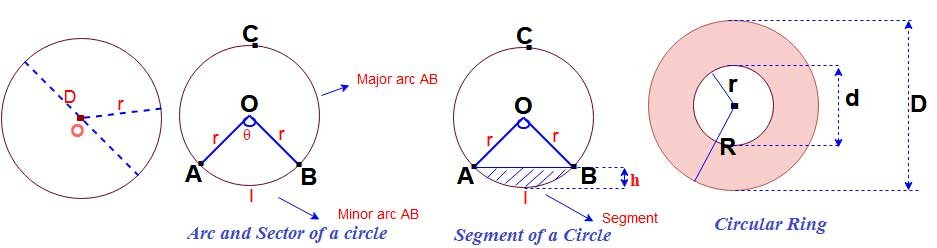#### Formulas for Area and circumference of the circle

Area of a circle (A ) =  π x (Radius) 2   =  π r 2 = ( π/4 ) D2 = 0.7854 x D2

Diameter of a circle (D) =Circumference of a circle ( C ) = 2 π x Radius =  2 π r = π D

Area of circle =( 1/2) x Circumference x radius = (1/2) x C x  r

#### Formula for Arc and sector of a circle

Arc length  of circle ( l ) =Area of the sector (minor) =If the angle θ is in radians mean   one radian = 180/π  , then

The area of the sector =Sector angle of a circle θ =#### Segment of circle and perimeter of segment

Area of the segment =Perimeter of the segment =Arc  Length of the circle segment   =  l  = 0.01745 x r x θ

Chord length of the circle ==#### Area of the circular ring

Area of a circular ring  = = (π/4)  ( D 2 – d 2)   = 0.7854 (D 2 – d 2)

Area of a circular ring  = π (R 2 – r 2 )

### Ellipse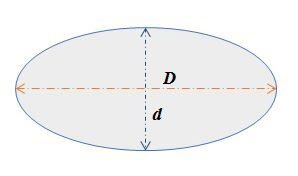D = Diameter of higher half-axis & d = Diameter of lower half-axis

Area of an Ellipse == 0.7854 x D x d

D = 2 x radius = 2 m , d = 2 x radius = 2 n

Perimeter of an Ellipse (Formula – 1)Perimeter of an Ellipse (Formula – 2)Both formulas gives approximate values but the formula- 2 gives better approch which is derived by Indian mathematician Ramanujan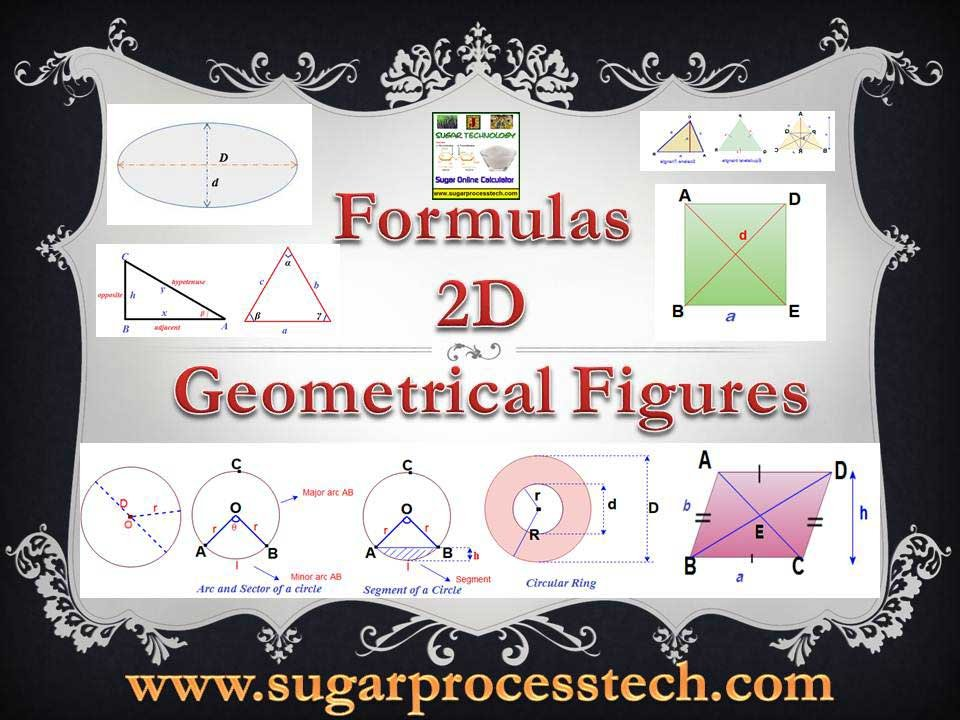Related Articles:

Conversion of units for Engineering Equipment Design & Drawing Calculations

Sugar Industry Manufacturing Process Machinery Capacity Calculation

## 2 thoughts on “Perimeter and Area Formulas for two dimensional geometrical figures”#### Pankaj kumar

(November 20, 2022 - 3:43 pm)

Good#### siva alluri

(December 2, 2022 - 2:44 pm)

welcome